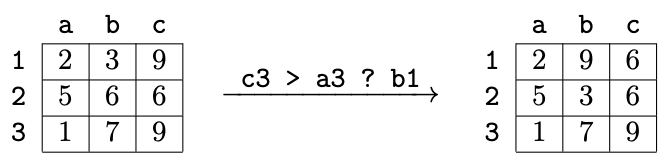시간 제한 메모리 제한 제출 정답 맞은 사람 정답 비율
1 초 512 MB 1 0 0 0.000%

## 문제

A robot can execute a sequence of commands in an $n \times n$ square filled with numbers. The only type of command the robot can execute is:

[cell 1] > [cell 2] ? [cell 3]

Such command has the following effect: if the number in cell 1 is greater than the number in cell 2, then rotate counterclockwise a $2 \times 2$ square whose upper left corner is cell 3.

Each cell is described with a letter denoting its column and a digit denoting its row, concatenated without a space. The columns are marked from left to right staring with a, the rows are marked from top to bottom starting with 1.

If one of the cells in one of the commands doesn't fit into the $n \times n$ square, or if cell 3 is located in the bottommost row or the rightmost column, then the robot becomes broken.An example of a single command execution. The condition is true ($9 > 1$), therefore the rotation is made.

After the execution of the entire program, the bottommost $n-2$ rows are written down concatenated in the order from top to bottom (the 3-rd row, the 4-th row, $\ldots$, the $n$-th row). The resulting sequence consisting of $n\cdot(n-2)$ numbers must be non-decreasing.

You are given an integer $n$. Output a program containing only commands of the described type, such that when a robot executes this program on any initial square with numbers, it doesn't become broken and makes the square satisfy the desired state.

## 입력

The first line contains a positive integer $n$ ($2 \le n \le 9$) --- the size of the square with numbers.

## 출력

Output a correct program. It must contain no more than $100\,000$ commands. Follow the output format shown in the example.

## 예제 입력 1

2


## 예제 출력 1

a2 > b2 ? a1
a2 > b2 ? a1
a2 > b2 ? a1


## 힌트

In order not to give a hint to the contestants, an example for $n \ge 3$ is not shown.

For $n = 2$ it is enough to obtain 0 non-decreasing rows, thus any syntactically correct program is a correct solution. But the shown program obtains not just 0, but 1 non-decreasing row in the bottom part of the square: it rotates the only $2 \times 2$ square counterclockwise until its bottom row becomes sorted non-decreasingly. It easy to prove that such situation will happen after 0, 1, 2 or 3 rotations.